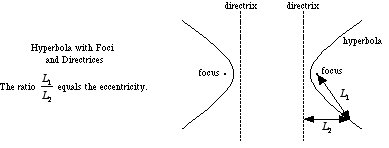index: click on a letter A B C D E F G H I J K L M N O P Q R S T U V W X Y Z A to Z index index: subject areas numbers & symbols sets, logic, proofs geometry algebra trigonometry advanced algebra & pre-calculus calculus advanced topics probability & statistics real world applications multimedia entrieswww.mathwords.com about mathwords website feedback

 Directrices of a Hyperbola Two parallel lines which are perpendicular to the major axis of a hyperbola. The directrices are between the two parts of a hyperbola and can be used to define it as follows: A hyperbola is the locus of points such that the ratio of the distance to the nearer focus to the distance to the nearer directrix equals a constant that is greater than one. This constant is the eccentricity.See also